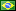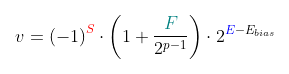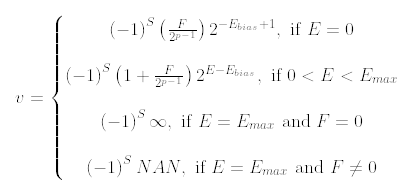###Author Topic: What is "floating point"?  (Read 511 times)

####fredericopissarra

• Full Member
•• Posts: 352
• Country:##### What is "floating point"?
« on: June 22, 2023, 02:42:42 PM »
There are two ways to represent values between 0 and 1: Using the positional notation, where a "point" divides the value in integer/fractional part and a fraction between 2 integer values. 0.5 means 5/10 of a unit, as an example.

The "floating" part means the point can go to the left or right if you multiply a fraction by a "scale factor", like 3.0 * 10^-2 can be writen making the point float to the left 2 positions: 0.03.

Positional notation is valid in binary. 0b0.01 is the same as 2^-2.

This way all "floating point" values are values between 1 and 2, excluding 2, multiplied by a power of 2 (the "scale factor" which makes the point float). The structuire of a single precision floating point value is this:Where the 3 parts are always unsigned integers. This structure shows the fractional part (F) with 23 bits, the expoent of the "scale factor" with 8 bits and a single signal bit (1=negative, 0=positive). Using this format, fractional values can be expressed by this equation:Where p=24, F has 23 bits, E has 8 bits e Ebias=127 (for float). For double, p=53, F has 52 bits and E is 11 bits long (Ebias=1023).

Notice in the figure of the structure above (taken from wikipedia), F=2^21=2097152 and E=124. This gives us v=(1+2097152/8388608)*2^-3=0.15625.

Values where 0<E<Emax are "normalized", since the integral part, before the scaling, is always 1. When E=0 we have a "sub-normal", where the integral part is always 0 and the scale factor is 2^(-Ebias+1). This way, 0.0 is expressed having E=0 and F=0 and.

It is obvious that the domain of these values are not in R, but in Q, because "floating point" structure can accomodate only proper fractions (where the numerator is always less the denominator), scaled.

Again, the middle part (1+F/2^23) is always a value between 1 and 2 (excluding 2) if 0<E<Emax. This way, if you have a decimal value and want to convert the a float structure, all you have to do is to discover the scale of that number and find E; rescale the value and find F:

e = floor(log2(v)); E=e+127. (if E<=0, E=0 and the value is sub-normal).
F(normal)=(v/(2^e) - 1)*2^23. (rounding required)

Since the value unscaled is always between 1 and 2, taking 0.15625 as an example, the actual value must be between 2^-3 and 2^-4, or 0.125 and 0.25, so we must rescale the value to get F, stripping the integral part (1). Makes sense to calculate the scale factor first, don't you think?

Here's another way to do it: Lets convert 3.1415 to float. In binary 3 is 0b11, but what about 0.1415? Ignoring the ingral part we keep multiplying by 2 until get 0.0 or reach the end of the type precision. 0.1415 can be converted to binary as:
Code: [Select]
`0.1415 * 2 = 0.283 (result in first position is 0)0.283 * 2 = 0.566 (result 0)0.566 * 2 = 1.132 (result 1 - next pass, ignore integral part)0.132 * 2 = 0.264 (result 0)0.264 * 2 = 0.528 (0)0.527 * 2 = 1.056 (1)...`We'll get 0b0.001001000011100101011000000100000110001001001101110100101111... So, 3.1415 is 0b11.00100100001110010101100... But, since the value have just 1 as the integral part, the point must float 1 position to the left. rescaling it by 2^1. So, 3.1415 is 0b1.10010010000111001010110_0 times 2, where this final 0 tells us that F dont't need to be rounded up (because F must have only 23 bits for a float) and we get 0b1.10010010000111001010110 * 2^1.

Here F=4787798, E=126 and S=0. Using the original equation: v=(1+4787798/8388608)*2^1=3.141499996185302734375

We can test this in C:
Code: [Select]
`#include <stdio.h>int main( void ){  float f = 3.1415f;  unsigned int *p = (unsigned int *)&f;  printf( "%f -> 0x%08x\n", f, *p );}`And we'll get:
Code: [Select]
`3.141500 -> 0x40490e56`0x40490e56 is 0b0_10000000_10010010000111001010110. Or F=4787798, E=128 and S=0, qed.
« Last Edit: June 22, 2023, 03:32:10 PM by fredericopissarra »

####fredericopissarra

• Full Member
•• Posts: 352
• Country:##### Re: What is "floating point"?
« Reply #1 on: June 22, 2023, 03:25:22 PM »
Ahhh... I cheat a little. To convert a fraction value to binary I use `bc`:
Code: [Select]
`\$ bcobase=23.141500000000011.00100100001110010101100000010000011000100100`Adding 0's to the right will increase the binary "precision"...

Multiplying by 2 the fraction decimal part ignoring the integral part works, but bc do this for me!####fredericopissarra

• Full Member
•• Posts: 352
• Country:##### Re: What is "floating point"?
« Reply #2 on: June 22, 2023, 04:04:31 PM »
To the curious, the complete equation for the floating point structure is:« Last Edit: June 22, 2023, 04:13:40 PM by fredericopissarra »